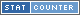# Calculate: 0 ^ 2 = 0

## How do you solve 0 ^ 2?

• Step #1 0^2 = 0

## Word Phrase for 0 ^ 2 = 0

Internationalization (i18n) word phrase of the math problem 0 ^ 2 = 0

• English (EN): zero to the power of two equals zero
• Spanish (ES): cero a la potencia de dos es igual a cero
• French (FR): zйro √† la puissance de deux √©gaux zйro
• German (DE): null hoch zwei ist gleich null
• Italian (IT): zero alla potenza di due uguale zero
• Indonesian (ID): nol untuk kekuagaris singgung dua sama nol
• Russian (RU): ноль –Ї –≤–ї–∞—Б—В–Є два —А–∞–≤–љ–Њ ноль
• Swedish (SV): noll till makt tvе lika noll
• Turkish (TR): s?f?r g√Љc√Љne e≈Яittir s?f?r

Q: Is the solution a whole number?
A: Yes, 0 is a whole number.

Q: Is the answer a positive or negative number?
A:0 (zero) is unigned, so it is neither positive nor negative.

## Solve in Base Systems

The equation 0 ^ 2 = 0 is represented in base 10 above. Here we show the same calculation but represented in other base counting systems. A base counting system is how many numbers are represented as group before advancing to the next digit. Example we normally use base 10 with numbers 0 to 9. When we add a 1 to the number 9 it becomes 10. For a base 3 system when a 1 is added to 2, it does not become 3 it becomes 10.

 Base Base Equation Base Answer 2 (binary) 0 ^ 10 0 3 0 ^ 2 0

## Simular problems to 0 ^ 2 = 0